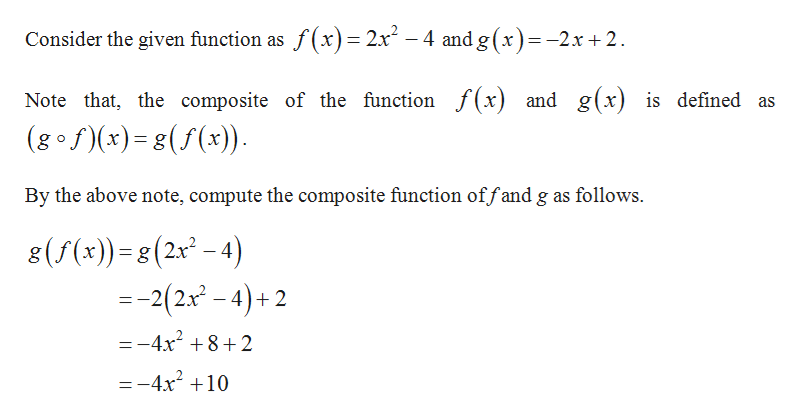# Givenf(x) = 2x2 − 4andg(x) = −2x + 2,find the following. gf (−3)

Question
39 views

Given

f(x) = 2x2 − 4

and

g(x) = −2x + 2,

find the following.

 g f
(−3)
check_circle

Step 1help_outlineImage TranscriptioncloseConsider the given function as f(x)= 2x -4 and g (x)= -2x + 2 Note that, the composite of the function f(x) and g(x) is defined as (gof(x)g(f(x)) By the above note, compute the composite function offand g as follows 8(f(x))- 8 (2x-4) --2(2x -4)+2 g =-4x2 8+2 = -4x2 10 fullscreen

### Want to see the full answer?

See Solution

#### Want to see this answer and more?

Solutions are written by subject experts who are available 24/7. Questions are typically answered within 1 hour.*

See Solution
*Response times may vary by subject and question.
Tagged in

### Other